# Solving Inequalities by Multiplying or Dividing Warm Up

• Slides: 20
Download presentation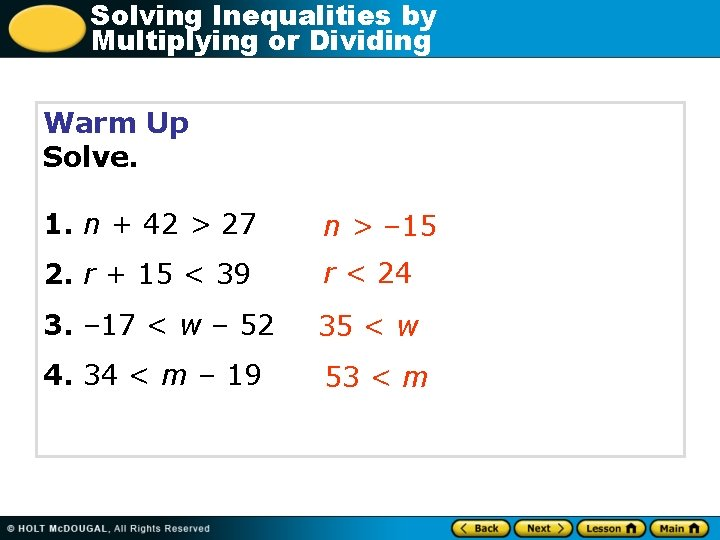Solving Inequalities by Multiplying or Dividing Warm Up Solve. 1. n + 42 > 27 n > – 15 2. r + 15 < 39 r < 24 3. – 17 < w – 52 35 < w 4. 34 < m – 19 53 < m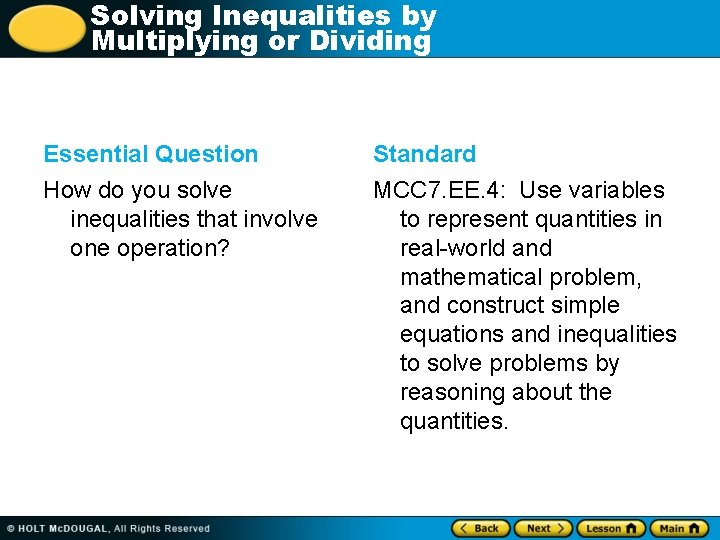Solving Inequalities by Multiplying or Dividing Essential Question Standard How do you solve inequalities that involve one operation? MCC 7. EE. 4: Use variables to represent quantities in real-world and mathematical problem, and construct simple equations and inequalities to solve problems by reasoning about the quantities.Solving Inequalities by Multiplying or DividingSolving Inequalities by Multiplying or Dividing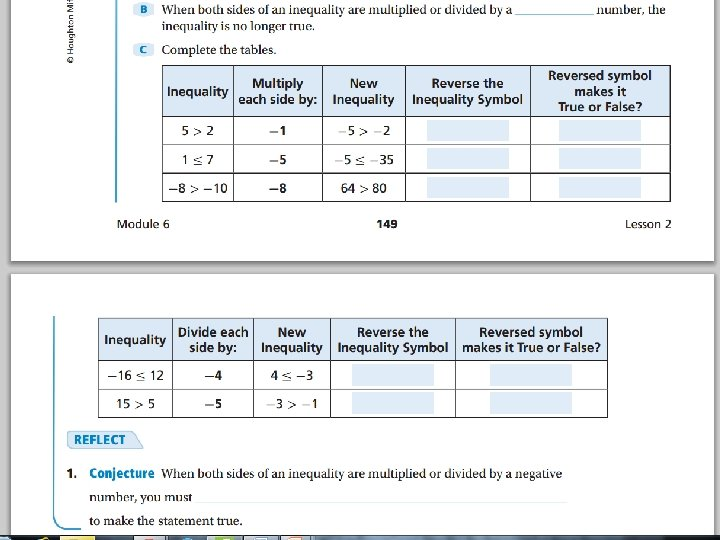Solving Inequalities by Multiplying or DividingSolving Inequalities by Fill in notes then glue into interactive Multiplying or Dividing notebook. -3 y = 9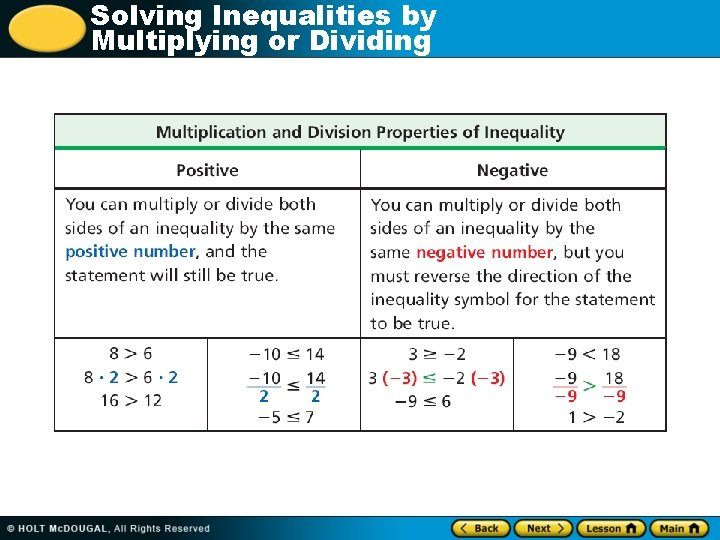Solving Inequalities by Multiplying or DividingSolving Inequalities by Multiplying or Dividing Fold example note page in ½ hotdog style. Label one side FLIP and the other DON’T FLIP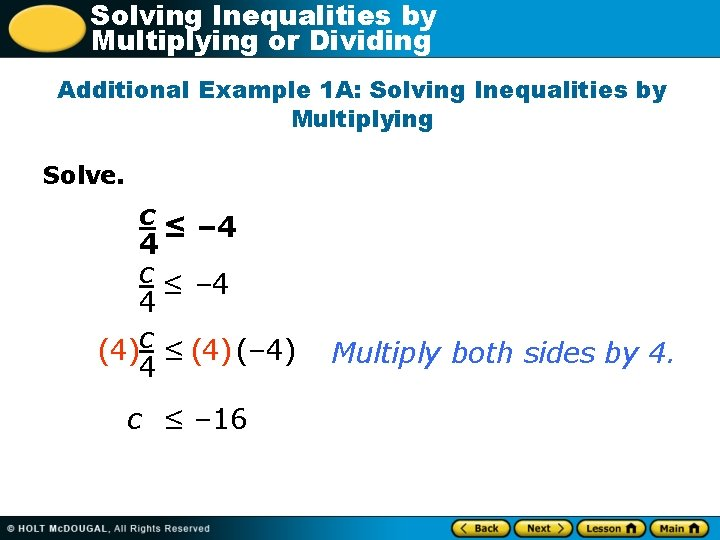Solving Inequalities by Multiplying or Dividing Additional Example 1 A: Solving Inequalities by Multiplying Solve. c ≤ – 4 4 (4)c ≤ (4) (– 4) 4 c ≤ – 16 Multiply both sides by 4.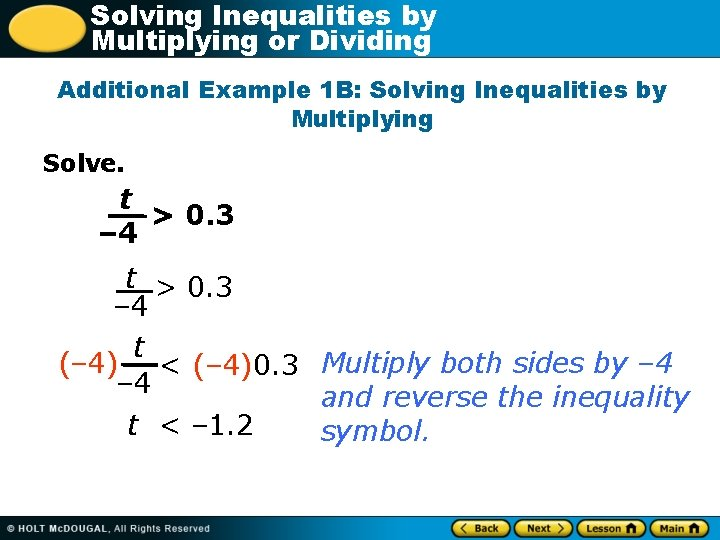Solving Inequalities by Multiplying or Dividing Additional Example 1 B: Solving Inequalities by Multiplying Solve. t > 0. 3 – 4 t (– 4) < (– 4)0. 3 Multiply both sides by – 4 and reverse the inequality t < – 1. 2 symbol.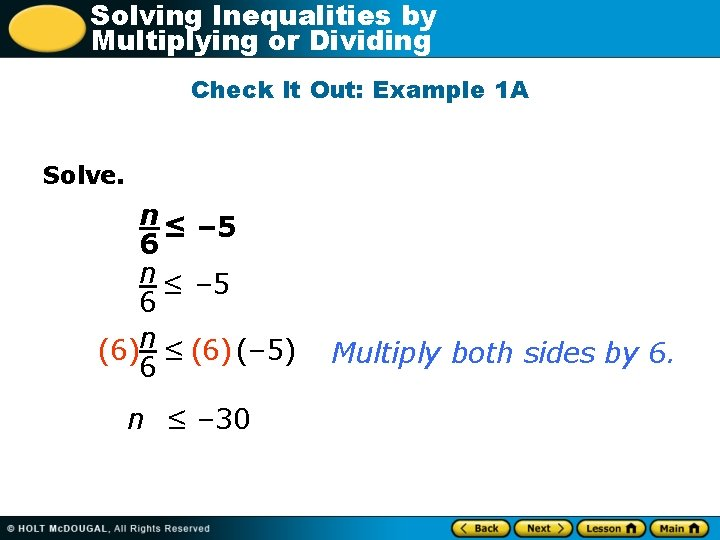Solving Inequalities by Multiplying or Dividing Check It Out: Example 1 A Solve. n ≤ – 5 6 (6)n ≤ (6) (– 5) 6 n ≤ – 30 Multiply both sides by 6.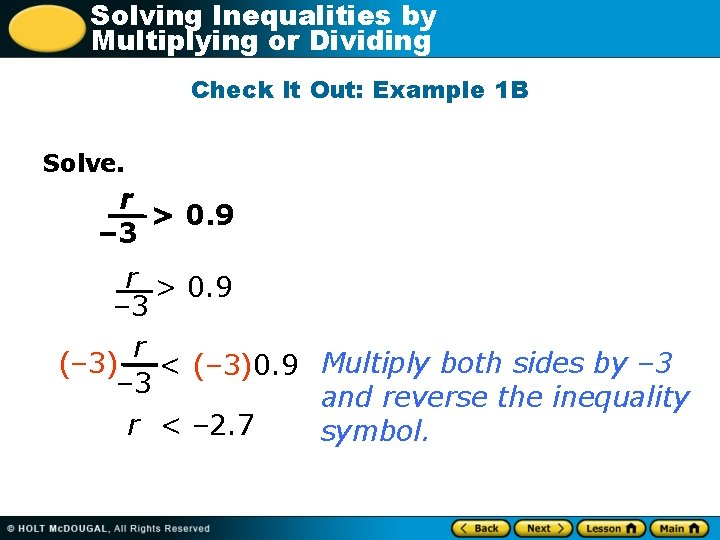Solving Inequalities by Multiplying or Dividing Check It Out: Example 1 B Solve. r > 0. 9 – 3 r (– 3) < (– 3)0. 9 Multiply both sides by – 3 and reverse the inequality r < – 2. 7 symbol.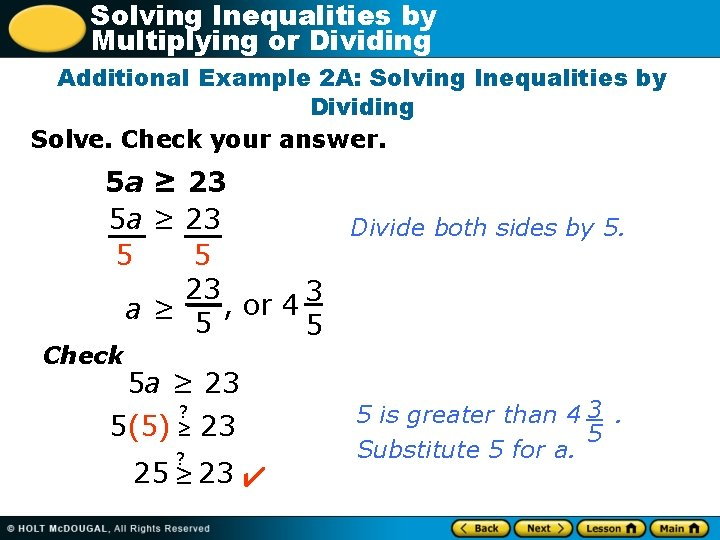Solving Inequalities by Multiplying or Dividing Additional Example 2 A: Solving Inequalities by Dividing Solve. Check your answer. 5 a ≥ 23 Divide both sides by 5. 5 5 23 3 , or 4 a≥ 5 5 Check 5 a ≥ 23 ? 5(5) ≥ 23 ? 25 ≥ 23 5 is greater than 4 3. 5 Substitute 5 for a.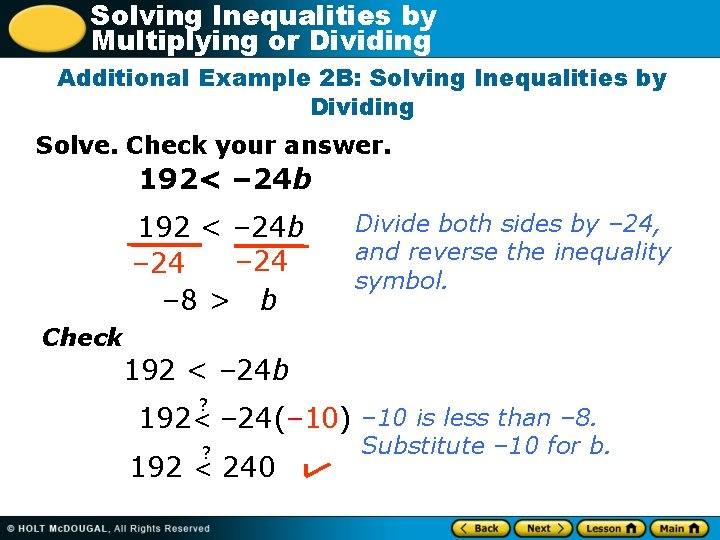Solving Inequalities by Multiplying or Dividing Additional Example 2 B: Solving Inequalities by Dividing Solve. Check your answer. 192< – 24 b 192 < – 24 b – 24 – 8 > b Divide both sides by – 24, and reverse the inequality symbol. Check 192 < – 24 b ? 192< – 24(– 10) – 10 is less than – 8. ? 192 < 240 Substitute – 10 for b.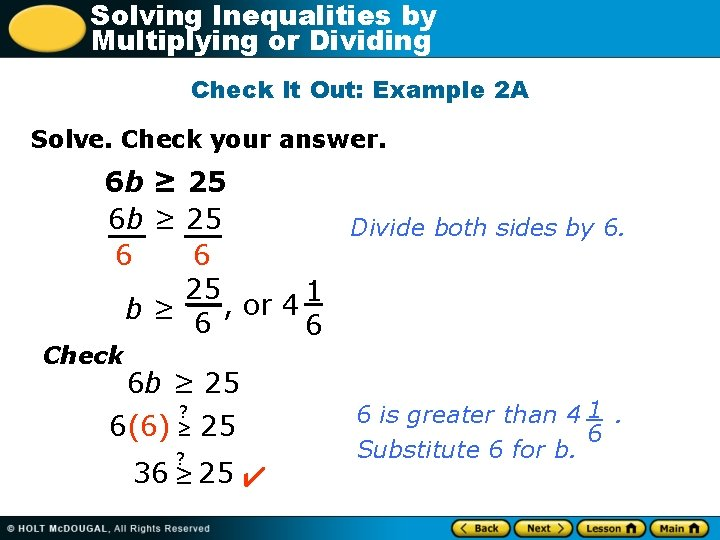Solving Inequalities by Multiplying or Dividing Check It Out: Example 2 A Solve. Check your answer. 6 b ≥ 25 Divide both sides by 6. 6 6 25 1 , or 4 b≥ 6 6 Check 6 b ≥ 25 ? 6(6) ≥ 25 ? 36 ≥ 25 6 is greater than 4 1. 6 Substitute 6 for b.Solving Inequalities by Multiplying or Dividing Check It Out: Example 2 B Solve. Check your answer. 85 < – 17 b – 17 – 5 > b Divide both sides by – 17, and reverse the inequality symbol. Check 85 < – 17 b ? 85 < – 17(– 6) – 6 is less than – 5. ? 85 < 102 Substitute – 6 for b.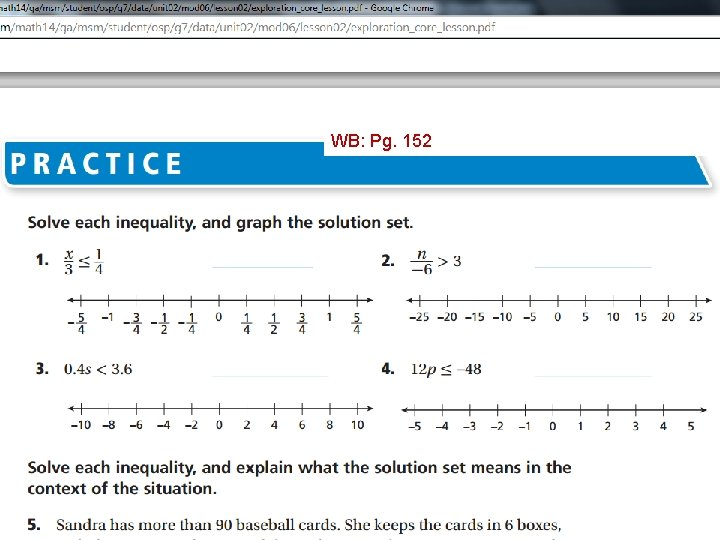Solving Inequalities by Multiplying or Dividing WB: Pg. 152Solving Inequalities by Multiplying or Dividing Homework: Workbook Pg. 153 #1 -12 Choose 3 division and 3 multiplication problemsSolving Inequalities by Multiplying or Dividing Check your homework answers: Pg. 153 1. 2. 3. 4. 5. 6. n<8 b > -24 a > -27 t > 42 s > 60 r < 31. 8 Review Video Class work: Inequality worksheet 7. c < - 8 8. a < 1. 5 9. t < -3/4 10. s > 60 11. b > -1 1/3 12. m < -2/3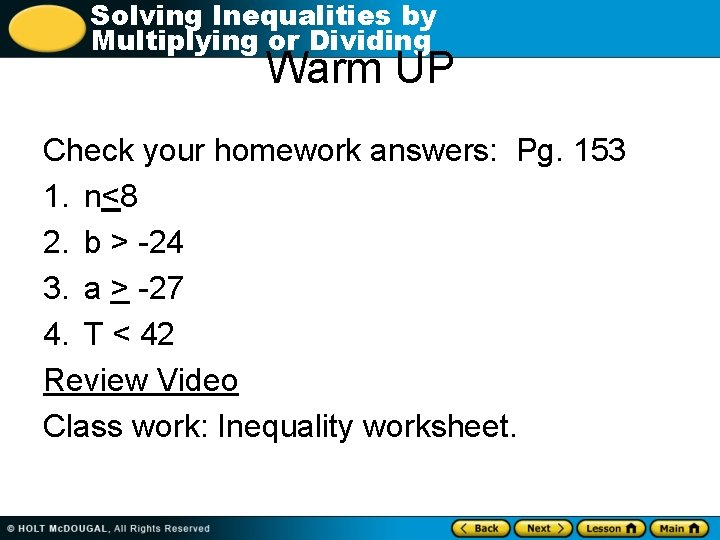Solving Inequalities by Multiplying or Dividing Warm UP Check your homework answers: Pg. 153 1. n<8 2. b > -24 3. a > -27 4. T < 42 Review Video Class work: Inequality worksheet.